## Machine Learning Stock Market Prediction with LSTM Keras

In the previous posts [1,2] I created script for machine learning stock market price on next day prediction. But it was pointed by readers that in stock market prediction, it is more important to know the trend: will the stock go up or down. So I updated the script to predict difference between today and yesterday prices. If it is negative the stock price will go down, if positive it will go up. Below will be described implemented modifications.

## Data inputting

Data from previous days are entered as features through additional columns. The number of columns can be changed through parameter N in the beginning of script. So for example for day 20 the input will contain data for day 21 as target and data for days 20, 19,18,17… 20-N
Also added differencing before scaling. Differencing helped to improve performance of network. It also makes easy to get changes from previous day. In the end the differenced data inverted back.

Below is the stock data prices after applying differencing (subtructing previous day stock data price from current day)Stock Data Prices after Differencing

## Predicting future changes

I used moving_test_window_preds function. Inside of this function the script within loop is adding new prediction to “moving window” array and removing first element from it. This is based on example from blog post on forecasting time series with LSTM.
So the script is predicting future day data based on the previous known data in the “moving window”, updating known data and starting again. The performance evaluated by comparing predicted data with test (not used before) data.

## LSTM Configuration

The LSTM network is constructed as following:

```model = Sequential ()
input_shape =(len(cols), 1) ))
model.add (LSTM ( 400,  activation = 'relu', inner_activation = 'hard_sigmoid' , bias_regularizer=L1L2(l1=0.01, l2=0.01),  input_shape =(len(cols), 1), return_sequences = False ))
model.add(Dropout(0.3))
from keras import optimizers
model.add (Dense (output_dim =1, activation = 'linear', activity_regularizer=regularizers.l1(0.01)))
adam=optimizers.Adam(lr=0.01, beta_1=0.89, beta_2=0.999, epsilon=None, decay=0.0, amsgrad=True)
model.compile (loss ="mean_squared_error" , optimizer = "adam")
history=model.fit (x_train, y_train, batch_size =1, nb_epoch =1400, shuffle = False, validation_split=0.15)
```

Weight regularization together with differencing helped to decrease overfitting.

## Results

Performance of NN is 88% : 8 correct data out of 9. Below are the data charts that comparing predicted data (9 first days from 22 total days) with actual test data. Below you can find output chart and full python source code.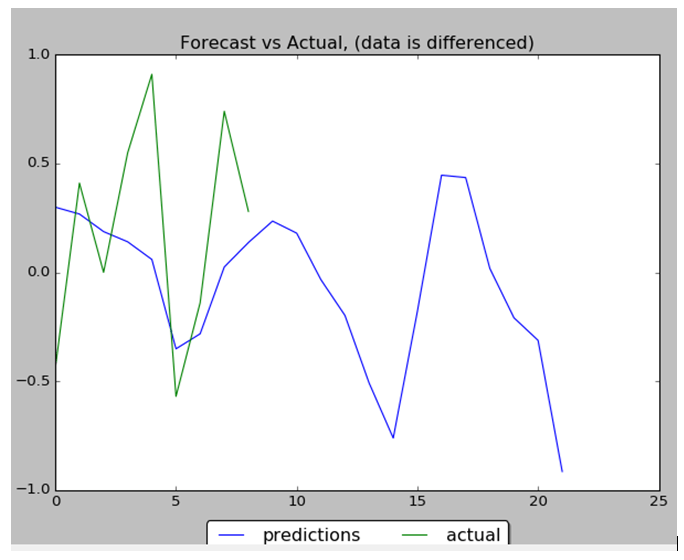Stock Data Prices Prediction with LSTM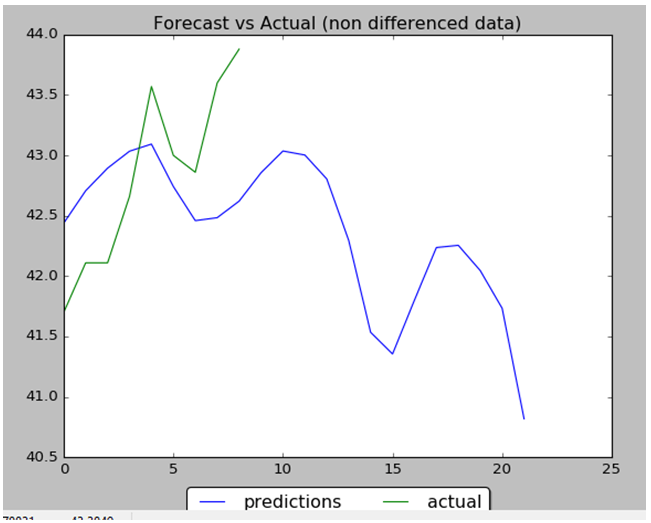Stock Data Prices Prediction with LSTM, Data Inverted Back from Differencing
```import numpy as np
import pandas as pd
from sklearn import preprocessing

import matplotlib.pyplot as plt
import matplotlib.ticker as mtick

from keras.regularizers import L1L2

fname="C:\\Users\\Leo\\Desktop\\A\\WS\\stock data analysis 2017\\GM.csv"
data_csv = pd.read_csv (fname)

#how many data we will use
# (should not be more than dataset length )
data_to_use= 150

# number of training data
# should be less than data_to_use
train_end =120

total_data=len(data_csv)

#most recent data is in the end
#so need offset
start=total_data - data_to_use

yt = data_csv.iloc [start:total_data ,4]    #Close price
yt_ = yt.shift (-1)

print (yt_)

data = pd.concat ([yt, yt_], axis =1)
data. columns = ['yt', 'yt_']

N=18
cols =['yt']
for i in range (N):

data['yt'+str(i)] = list(yt.shift(i+1))
cols.append ('yt'+str(i))

data = data.dropna()
data_original = data
data=data.diff()
data = data.dropna()

# target variable - closed price
# after shifting
y = data ['yt_']
x = data [cols]

scaler_x = preprocessing.MinMaxScaler ( feature_range =( -1, 1))
x = np. array (x).reshape ((len( x) ,len(cols)))
x = scaler_x.fit_transform (x)

scaler_y = preprocessing. MinMaxScaler ( feature_range =( -1, 1))
y = np.array (y).reshape ((len( y), 1))
y = scaler_y.fit_transform (y)

x_train = x [0: train_end,]
x_test = x[ train_end +1:len(x),]
y_train = y [0: train_end]
y_test = y[ train_end +1:len(y)]

x_train = x_train.reshape (x_train. shape + (1,))
x_test = x_test.reshape (x_test. shape + (1,))

from keras.models import Sequential
from keras.layers.core import Dense
from keras.layers.recurrent import LSTM
from keras.layers import  Dropout
from keras import optimizers

from numpy.random import seed
seed(1)
from tensorflow import set_random_seed
set_random_seed(2)

from keras import regularizers

model = Sequential ()
model.add (LSTM ( 400,  activation = 'relu', inner_activation = 'hard_sigmoid' , bias_regularizer=L1L2(l1=0.01, l2=0.01),  input_shape =(len(cols), 1), return_sequences = False ))
model.add(Dropout(0.3))
model.add (Dense (output_dim =1, activation = 'linear', activity_regularizer=regularizers.l1(0.01)))
adam=optimizers.Adam(lr=0.01, beta_1=0.89, beta_2=0.999, epsilon=None, decay=0.0, amsgrad=True)
model.compile (loss ="mean_squared_error" , optimizer = "adam")
history=model.fit (x_train, y_train, batch_size =1, nb_epoch =1400, shuffle = False, validation_split=0.15)

y_train_back=scaler_y.inverse_transform (np. array (y_train). reshape ((len( y_train), 1)))
plt.figure(1)
plt.plot (y_train_back)

fmt = '%.1f'
tick = mtick.FormatStrFormatter(fmt)
ax = plt.axes()
ax.yaxis.set_major_formatter(tick)
print (model.summary())
print(history.history.keys())

plt.figure(2)
plt.plot(history.history['loss'])
plt.plot(history.history['val_loss'])
plt.title('model loss')
plt.ylabel('loss')
plt.xlabel('epoch')
plt.legend(['train', 'test'], loc='upper left')
fmt = '%.1f'
tick = mtick.FormatStrFormatter(fmt)
ax = plt.axes()
ax.yaxis.set_major_formatter(tick)

score_train = model.evaluate (x_train, y_train, batch_size =1)
score_test = model.evaluate (x_test, y_test, batch_size =1)
print (" in train MSE = ", round( score_train ,4))
print (" in test MSE = ", score_test )

pred1 = model.predict (x_test)
pred1 = scaler_y.inverse_transform (np. array (pred1). reshape ((len( pred1), 1)))

prediction_data = pred1[-1]
model.summary()
print ("Inputs: {}".format(model.input_shape))
print ("Outputs: {}".format(model.output_shape))
print ("Actual input: {}".format(x_test.shape))
print ("Actual output: {}".format(y_test.shape))

print ("prediction data:")
print (prediction_data)

y_test = scaler_y.inverse_transform (np. array (y_test). reshape ((len( y_test), 1)))
print ("y_test:")
print (y_test)

act_data = np.array([row for row in y_test])

fmt = '%.1f'
tick = mtick.FormatStrFormatter(fmt)
ax = plt.axes()
ax.yaxis.set_major_formatter(tick)

plt.figure(3)
plt.plot( y_test, label="actual")
plt.plot(pred1, label="predictions")

print ("act_data:")
print (act_data)

print ("pred1:")
print (pred1)

plt.legend(loc='upper center', bbox_to_anchor=(0.5, -0.05),
fancybox=True, shadow=True, ncol=2)

fmt = '\$%.1f'
tick = mtick.FormatStrFormatter(fmt)
ax = plt.axes()
ax.yaxis.set_major_formatter(tick)

def moving_test_window_preds(n_future_preds):

''' n_future_preds - Represents the number of future predictions we want to make
This coincides with the number of windows that we will move forward
on the test data
'''
preds_moving = []                                    # Store the prediction made on each test window
moving_test_window = [x_test[0,:].tolist()]          # First test window
moving_test_window = np.array(moving_test_window)

for i in range(n_future_preds):

preds_one_step = model.predict(moving_test_window)
preds_moving.append(preds_one_step[0,0])

preds_one_step = preds_one_step.reshape(1,1,1)
moving_test_window = np.concatenate((moving_test_window[:,1:,:], preds_one_step), axis=1) # new moving test window, where the first element from the window has been removed and the prediction  has been appended to the end

print ("pred moving before scaling:")
print (preds_moving)

preds_moving = scaler_y.inverse_transform((np.array(preds_moving)).reshape(-1, 1))

print ("pred moving after scaling:")
print (preds_moving)
return preds_moving

print ("do moving test predictions for next 22 days:")
preds_moving = moving_test_window_preds(22)

count_correct=0
error =0
for i in range (len(y_test)):
error=error + ((y_test[i]-preds_moving[i])**2) / y_test[i]

if y_test[i] >=0 and preds_moving[i] >=0 :
count_correct=count_correct+1
if y_test[i] < 0 and preds_moving[i] < 0 :
count_correct=count_correct+1

accuracy_in_change =  count_correct / (len(y_test) )

plt.figure(4)
plt.title("Forecast vs Actual, (data is differenced)")
plt.plot(preds_moving, label="predictions")
plt.plot(y_test, label="actual")
plt.legend(loc='upper center', bbox_to_anchor=(0.5, -0.05),
fancybox=True, shadow=True, ncol=2)

print ("accuracy_in_change:")
print (accuracy_in_change)

ind=data_original.index.values + data_original.shape -len(y_test)-1
prev_starting_price = data_original.loc[ind,"yt_"]
preds_moving_before_diff =  [0 for x in range(len(preds_moving))]

for i in range (len(preds_moving)):
if (i==0):
preds_moving_before_diff[i]=prev_starting_price + preds_moving[i]
else:
preds_moving_before_diff[i]=preds_moving_before_diff[i-1]+preds_moving[i]

y_test_before_diff = [0 for x in range(len(y_test))]

for i in range (len(y_test)):
if (i==0):
y_test_before_diff[i]=prev_starting_price + y_test[i]
else:
y_test_before_diff[i]=y_test_before_diff[i-1]+y_test[i]

plt.figure(5)
plt.title("Forecast vs Actual (non differenced data)")
plt.plot(preds_moving_before_diff, label="predictions")
plt.plot(y_test_before_diff, label="actual")
plt.legend(loc='upper center', bbox_to_anchor=(0.5, -0.05),
fancybox=True, shadow=True, ncol=2)
plt.show()
```

## Time Series Prediction with LSTM and Keras for Multiple Steps Ahead

In this post I will share experiment with Time Series Prediction with LSTM and Keras. LSTM neural network is used in this experiment for multiple steps ahead for stock prices data. The experiment is based on the paper . The authors of the paper examine independent value prediction approach. With this approach a separate model is built for each prediction step. This approach helps to avoid error accumulation problem that we have when we use multi-stage step prediction.

### LSTM Implementation

Following this approach I decided to use Long Short-Term Memory network or LSTM network for daily data stock price prediction. LSTM is a type of recurrent neural network used in deep learning. LSTMs have been used to advance the state-of the-art for many difficult problems. 

For this time series prediction I selected the number of steps to predict ahead = 3 and built 3 LSTM models with Keras in python. For each model I used different variable (fit0, fit1, fit2) to avoid any “memory leakage” between models.
The model initialization code is the same for all 3 models except changing parameters (number of neurons in LSTM layer)
The architecture of the system is shown on the fig below.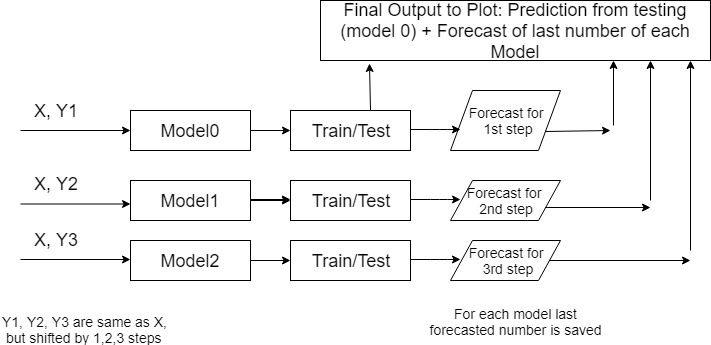Multiple step prediction with separate neural networks

Here we have 3 LSTM models that are getting same X input data but different target Y data. The target data is shifted by number of steps. If model is forecasting the data stock price for day 2 then Y is shifted by 2 elements.
This happens in the following line when i=1:

```yt_ = yt.shift (-i - 1  )
```

The data were obtained from stock prices from Internet.

The number of unit was obtained by running several variations and chosen based on MSE as following:

```
if i==0:
units=20
batch_size=1
if i==1:
units=15
batch_size=1
if i==2:
units=80
batch_size=1
```

If you want run more than 3 steps / models you will need to add parameters to the above code. Additionally you will need add model initialization code shown below.

Each LSTM network was constructed as following:

```
if i == 0 :
fit0 = Sequential ()
fit0.add (LSTM (  units , activation = 'tanh', inner_activation = 'hard_sigmoid' , input_shape =(len(cols), 1) ))
fit0.add(Dropout(0.2))
fit0.add (Dense (output_dim =1, activation = 'linear'))
fit0.compile (loss ="mean_squared_error" , optimizer = "adam")

fit0.fit (x_train, y_train, batch_size =batch_size, nb_epoch =25, shuffle = False)
train_mse[i] = fit0.evaluate (x_train, y_train, batch_size =batch_size)
test_mse[i] = fit0.evaluate (x_test, y_test, batch_size =batch_size)
pred = fit0.predict (x_test)
pred = scaler_y.inverse_transform (np. array (pred). reshape ((len( pred), 1)))
# below is just fo i == 0
for j in range (len(pred)) :
prediction_data[j] = pred[j]
```

For each model the code is saving last forecasted number.
Additionally at step i=0 predicted data is saved for comparison with actual data:

```prediction_data = np.asarray(prediction_data)
prediction_data = prediction_data.ravel()

# shift back by one step
for j in range (len(prediction_data) - 1 ):
prediction_data[len(prediction_data) - j - 1  ] =  prediction_data[len(prediction_data) - 1 - j - 1]

# combine prediction data from first model and last predicted data from each model
prediction_data = np.append(prediction_data, forecast)
```

The full python source code for time series prediction with LSTM in python is shown here

Data can be found here

### Experiment Results

The LSTM neural network was running with the following performance:

```train_mse
[0.01846262458218137, 0.009637593373373323, 0.0018845983509225203]
test_mse
[0.01648362025879952, 0.026161141224167357, 0.01774421124347165]
```

Below is the graph of actual data vs data testing data, including last 3 stock data prices from each model.Multiple step prediction – actual data vs predictions

Accuracy of prediction 98% calculated for last 3 data stock prices (one from each model).

The experiment confirmed that using models (one model for each step) in multistep-ahead time series prediction has advantages. With this method we can adjust parameters of needed LSTM for each step. For example, number of neurons for i=2 was modified to decrease prediction error for this step. And it did not affect predictions for other steps. This is one of machine learning techniques for stock prediction that is described in 

## Machine Learning Stock Prediction with LSTM and Keras

In this post I will share experiments on machine learning stock prediction with LSTM and Keras with one step ahead. I tried to do first multiple steps ahead with few techniques described in the papers on the web. But I discovered that I need fully understand and test the simplest model – prediction for one step ahead. So I put here the example of prediction of stock price data time series for one next step.

### Preparing Data for Neural Network Prediction

Our first task is feed the data into LSTM. Our data is stock price data time series that were downloaded from the web.
Our interest is closed price for the next day so target variable will be closed price but shifted to left (back) by one step.

Figure below is showing how do we prepare X, Y data.
Please note that I put X Y horizontally for convenience but still calling X, Y as columns.
Also on this figure only one variable (closed price) is showed for X but in the code it is actually more than one variable (it is closed price, open price, volume and high price).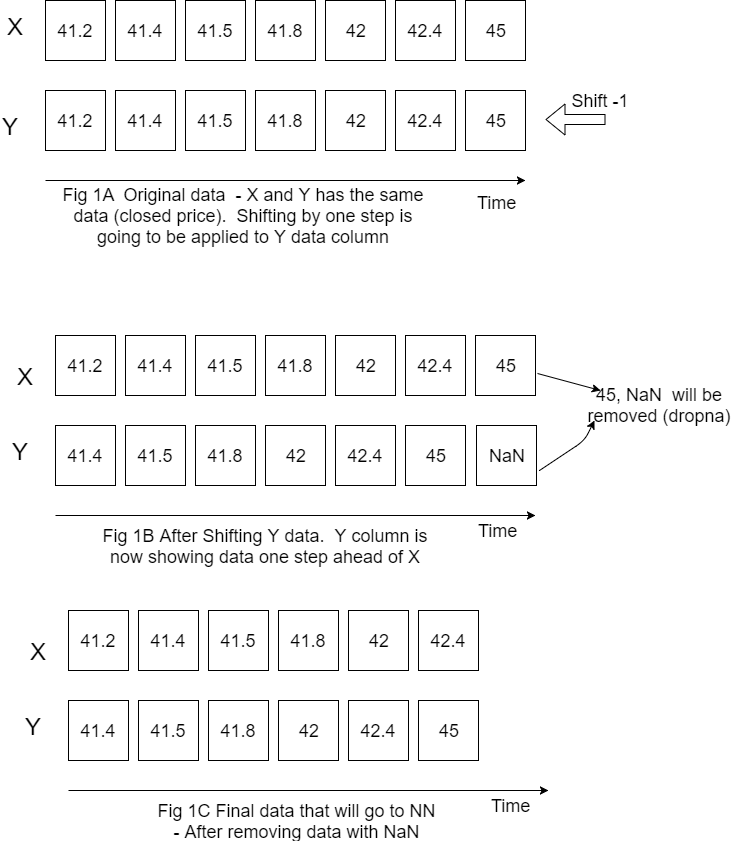LSTM data input

Now we have the data that we can feed for LSTM neural network prediction. Before doing this we need also do the following things:

1. Decide how many data we want to use. For example if we have data for 10 years but want use only last 200 rows of data we would need specify start point because the most recent data will be in the end.

```#how many data we will use
# (should not be more than dataset length )
data_to_use= 100

# number of training data
# should be less than data_to_use
train_end =70

total_data=len(data_csv)

#most recent data is in the end
#so need offset
start=total_data - data_to_use
```

2. Rescale (normalize) data as below. Here feature_range is tuple (min, max), default=(0, 1) is desired range of transformed data. 

```scaler_x = preprocessing.MinMaxScaler ( feature_range =( -1, 1))
x = np. array (x).reshape ((len( x) ,len(cols)))
x = scaler_x.fit_transform (x)

scaler_y = preprocessing. MinMaxScaler ( feature_range =( -1, 1))
y = np.array (y).reshape ((len( y), 1))
y = scaler_y.fit_transform (y)
```

3. Divide data into training and testing set.

```x_train = x [0: train_end,]
x_test = x[ train_end +1:len(x),]
y_train = y [0: train_end]
y_test = y[ train_end +1:len(y)]
```

### Building and Running LSTM

Now we need to define our LSTM neural network layers , parameters and run neural network to see how it works.

```fit1 = Sequential ()
fit1.add (LSTM (  1000 , activation = 'tanh', inner_activation = 'hard_sigmoid' , input_shape =(len(cols), 1) ))
fit1.add(Dropout(0.2))
fit1.add (Dense (output_dim =1, activation = 'linear'))

fit1.compile (loss ="mean_squared_error" , optimizer = "adam")
fit1.fit (x_train, y_train, batch_size =16, nb_epoch =25, shuffle = False)
```

The first run was not good – prediction was way below actual.

However by changing the number of neurons in LSTM neural network prediction code, it was possible to improve MSE and get predictions much closer to actual data. Below are screenshots of plots of prediction and actual data for LSTM with 5, 60 and 1000 neurons. Note that the plot is showing only testing data. Training data is not shown on the plots. Also you can find below the data for MSE and number of neurons. As the number neorons in LSTM layer is changing there were 2 minimums (one around 100 neurons and another around 1000 neurons)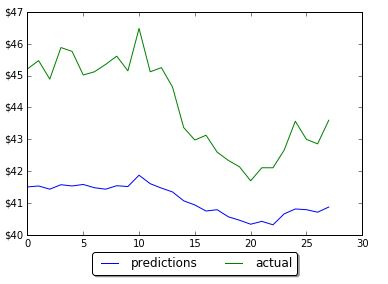Results of prediction on LSTM with 5 neurons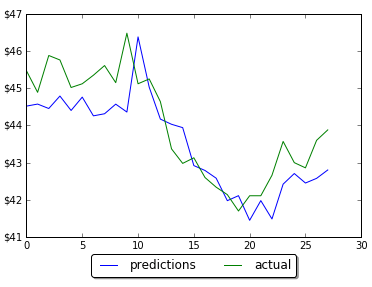Results of prediction on LSTM with 60 neurons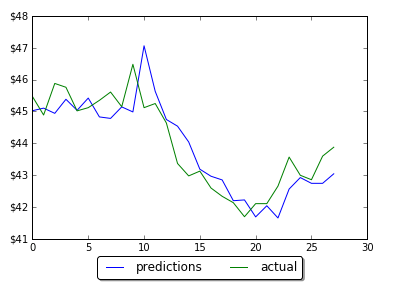Results of prediction on LSTM with 1000 neurons

LSTM 5
in train MSE = 0.0475
in test MSE = 0.2714831660102521

LSTM 60
in train MSE = 0.0127
in test MSE = 0.02227417068206705

LSTM 100
in train MSE = 0.0126
in test MSE = 0.018672733913263073

LSTM 200
in train MSE = 0.0133
in test MSE = 0.020082660237026824

LSTM 900
in train MSE = 0.0103
in test MSE = 0.015546535929778267

LSTM 1000
in train MSE = 0.0104
in test MSE = 0.015037054958075455

LSTM 1100
in train MSE = 0.0113
in test MSE = 0.016363980411369994

### Conclusion

The final LSTM was running with testing MSE 0.015 and accuracy 97%. This was obtained by changing number of neurons in LSTM. This example will serve as baseline for further possible improvements on machine learning stock prediction with LSTM. If you have any tips or anything else to add, please leave a comment the comment box. The full source code for LSTM neural network prediction can be found here